Contact Home

# Procedurally Generating Wrapping World Maps in Unity C# – Part 2

Check out Part 1 of this series if you haven’t already. This is a continuation of that article.

In the previous article (Part 1):

In Part 3:

In Part 4:

### Wrapping the Map on One Axis

In part 1 of this tutorial, we set up a nice little framework to help us build up our maps. The Height Map we created previously, was not tileable.

This is because we sampled 2D noise data, which is not capable of providing us with what we need. If we want to make our world wrap around seamlessly, then we are going to need to add some dimension to our noise generator.

With 3D noise, we can sample data in a circular pattern, and the resulting 2D data will wrap on a single axis. The sampled data would resemble a cylinder in 3D space.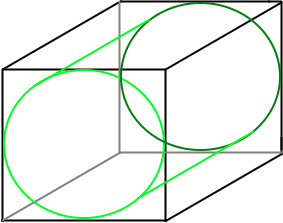Imagine if we took this cylinder, cut it open, and laid it flat. This is essentially what we will be doing. The ends where we made the cut, would be able to join together seamlessly.

In order to do this, we need to modify the GetData function in our Generator class.

```private void GetData(ImplicitModuleBase module, ref MapData mapData)
{
mapData = new MapData (Width, Height);

// loop through each x,y point - get height value
for (var x = 0; x < Width; x++) {
for (var y = 0; y < Height; y++) {

//Noise range
float x1 = 0, x2 = 1;
float y1 = 0, y2 = 1;
float dx = x2 - x1;
float dy = y2 - y1;

//Sample noise at smaller intervals
float s = x / (float)Width;
float t = y / (float)Height;

// Calculate our 3D coordinates
float nx = x1 + Mathf.Cos (s * 2 * Mathf.PI) * dx / (2 * Mathf.PI);
float ny = x1 + Mathf.Sin (s * 2 * Mathf.PI) * dx / (2 * Mathf.PI);
float nz = t;

float heightValue = (float)HeightMap.Get (nx, ny, nz);

// keep track of the max and min values found
if (heightValue > mapData.Max)
mapData.Max = heightValue;
if (heightValue < mapData.Min)
mapData.Min = heightValue;

mapData.Data [x, y] = heightValue;
}
}
}
```

Running this code, then gives us a nice texture, that wraps on the x-axis: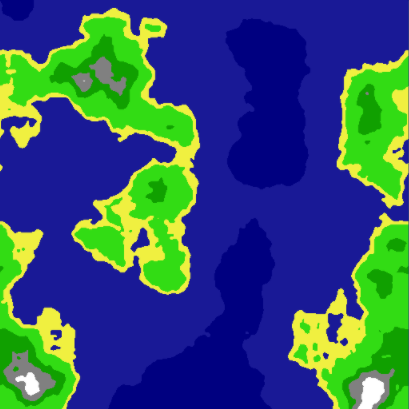### Wrapping the Map on Both Axis

In order to get our map to wrap around both axis, we need to start sampling 4D noise. This concept is a little more difficult to grasp, as our minds have a hard time thinking in 4 dimensions, but is very similar to the 3D example.

Instead of having a single cylinder, you would have two cylinders connected together, in a 4D space.

Keep in mind, that sampling 4D data takes a lot longer than sampling 2D data.

Our updated GetData() function would then look like this:

```private void GetData(ImplicitModuleBase module, ref MapData mapData)
{
mapData = new MapData (Width, Height);

// loop through each x,y point - get height value
for (var x = 0; x < Width; x++) {
for (var y = 0; y < Height; y++) {

// Noise range
float x1 = 0, x2 = 2;
float y1 = 0, y2 = 2;
float dx = x2 - x1;
float dy = y2 - y1;

// Sample noise at smaller intervals
float s = x / (float)Width;
float t = y / (float)Height;

// Calculate our 4D coordinates
float nx = x1 + Mathf.Cos (s*2*Mathf.PI) * dx/(2*Mathf.PI);
float ny = y1 + Mathf.Cos (t*2*Mathf.PI) * dy/(2*Mathf.PI);
float nz = x1 + Mathf.Sin (s*2*Mathf.PI) * dx/(2*Mathf.PI);
float nw = y1 + Mathf.Sin (t*2*Mathf.PI) * dy/(2*Mathf.PI);

float heightValue = (float)HeightMap.Get (nx, ny, nz, nw);

// keep track of the max and min values found
if (heightValue > mapData.Max) mapData.Max = heightValue;
if (heightValue < mapData.Min) mapData.Min = heightValue;

mapData.Data[x,y] = heightValue;
}
}
}
```

This code produces a seamless tileable texture, that is procedurally generated from 4D noise: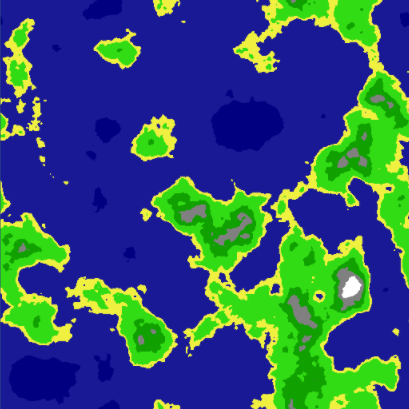If you would like more information on how this works, have a look here and here.

### Finding Neighbors

Now that we have a tileable Height Map, we are starting to get a lot closer to our goal. Now, we are going to shift focus towards the Tile class.

It would be very useful if each Tile object had a reference to each of its neighbors (top, bottom, left, right). This comes in handy for things, such as creating paths, bitmasking, or flood filling. We will touch on these aspects later on in this tutorial.

First thing we need to do, is create variables in our Tile class:

```public Tile Left;
public Tile Right;
public Tile Top;
public Tile Bottom;
```

The next part is pretty straightforward. We simply run through every single tile, setting it’s neighboring tiles. First off, we will create a few functions inside of our Generator class, to simplify retrieving the Tile neighbors:

```	private Tile GetTop(Tile t)
{
return Tiles [t.X, MathHelper.Mod (t.Y - 1, Height)];
}
private Tile GetBottom(Tile t)
{
return Tiles [t.X, MathHelper.Mod (t.Y + 1, Height)];
}
private Tile GetLeft(Tile t)
{
return Tiles [MathHelper.Mod(t.X - 1, Width), t.Y];
}
private Tile GetRight(Tile t)
{
return Tiles [MathHelper.Mod (t.X + 1, Width), t.Y];
}
```

MathHelper.Mod() will wrap the x and y values for us, based on our Map width and height. This will ensure we never go off of our map.

Next, we also need to add the function that will do the neighbor assignments:

```	private void UpdateNeighbors()
{
for (var x = 0; x < Width; x++)
{
for (var y = 0; y < Height; y++)
{
Tile t = Tiles[x,y];

t.Top = GetTop(t);
t.Bottom = GetBottom (t);
t.Left = GetLeft (t);
t.Right = GetRight (t);
}
}
}
```

Visually, this doesn’t do much, yet. However, each Tile now knows who their neighbors are, which is very important for future steps.

I decided to add this into the tutorial, mainly for aesthetic purposes. Bitmasking, in this context, is about assigning a value to each tile, based on it’s neighbors. Have a look at the following image: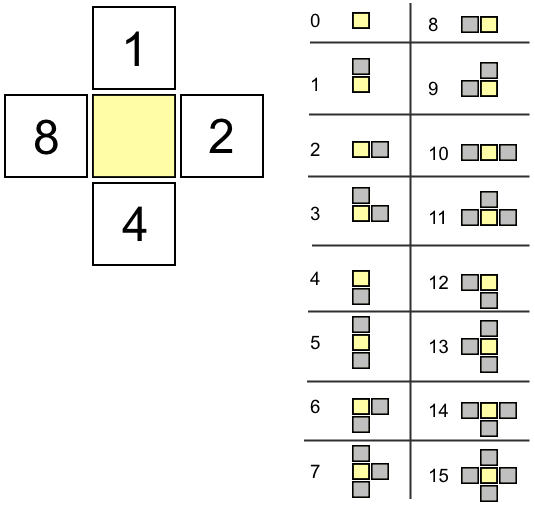Based on a tile’s neighbors, we increment the bitmask as shown on the left side of the above image. All possibilities are illustrated on the right side. Note that each value is unique. This allows us to identify a block’s configuration very quickly.

The main benefit of bitmasking, is that you can then assign a texture, based on the bitmask value of each tile, making your maps a lot prettier and way less blocky when done properly.

Another benefit of bitmasking, is that if a Tile’s bitmask value is not equal to 15, then we know it is an edge tile.

Let’s add a function in our Tile class to do the calculation. We are only concerned with neighbors that share the same Height Type as the tile being analyzed.

```	public void UpdateBitmask()
{
int count = 0;

if (Top.HeightType == HeightType)
count += 1;
if (Right.HeightType == HeightType)
count += 2;
if (Bottom.HeightType == HeightType)
count += 4;
if (Left.HeightType == HeightType)
count += 8;

}
```

Since we already have references to the neighboring tiles, and we also have defined a HeightType, this calculation is quite trivial. Next, we add a function in our Generator class, in order to process this calculation for all of the tiles:

```	private void UpdateBitmasks()
{
for (var x = 0; x < Width; x++) {
for (var y = 0; y < Height; y++) {
}
}
}
```

Now, if we modify our TextureGenerator as follows:

```//darken the color if a edge tile
pixels[x + y * width] = Color.Lerp(pixels[x + y * width], Color.black, 0.4f);

```

We can now see a defined edge between our Height Types: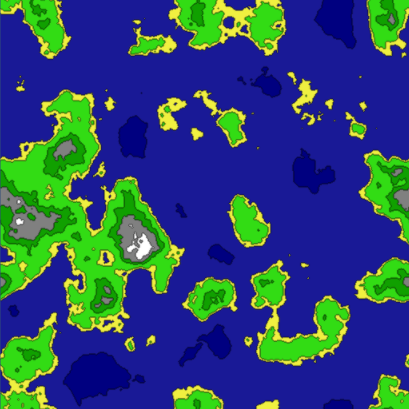### Flood Filling

It would be nice if we could determine a few things, such as:

• Where are the Lakes?
• Where are the Oceans?
• Where are the Land Masses?
• How big are each of these?

We can answer all of these question, with the help of a simple Flood Fill algorithm.

First, we are going to create an object, that will store information on our Tiles:

```using UnityEngine;
using System.Collections.Generic;

public enum TileGroupType
{
Water,
Land
}

public class TileGroup  {

public TileGroupType Type;
public List<Tile> Tiles;

public TileGroup()
{
Tiles = new List<Tile> ();
}
}
```

The TileGroup class will hold a reference to a list of Tiles. It will also let us know if this particular group is Water or Land.

The main idea is to break down connected pieces of land and water into TileGroup collections.

We are also going to modify the Tile class slightly by adding two new variables:

```	public bool Collidable;
public bool FloodFilled;
```

Collidable will be set inside of the LoadTiles() method. Anything that is not a water tile, will have Collidable set to true. The FloodFilled variable will be used to keep track of which tiles have already been processed by the flood filling algorithm.

In order to add our flood fill algorithm to the Generator class. First we are going to need a couple of TileGroup variables:

```	List<TileGroup> Waters = new List<TileGroup> ();
List<TileGroup> Lands = new List<TileGroup> ();
```

Now we are ready to determine land and water masses in our map.

Since the map could potentially be very large, we cannot use a recursive flood fill, as it would easily produce stack overflow exceptions. Instead, we will need to use a non-recursive approach to solve this problem:

```
private void FloodFill()
{
// Use a stack instead of recursion
Stack<Tile> stack = new Stack<Tile>();

for (int x = 0; x < Width; x++) {
for (int y = 0; y < Height; y++) {

Tile t = Tiles[x,y];

if (t.FloodFilled) continue;

// Land
if (t.Collidable)
{
TileGroup group = new TileGroup();
group.Type = TileGroupType.Land;
stack.Push(t);

while(stack.Count > 0) {
FloodFill(stack.Pop(), ref group, ref stack);
}

if (group.Tiles.Count > 0)
}
// Water
else {
TileGroup group = new TileGroup();
group.Type = TileGroupType.Water;
stack.Push(t);

while(stack.Count > 0)	{
FloodFill(stack.Pop(), ref group, ref stack);
}

if (group.Tiles.Count > 0)
}
}
}
}

private void FloodFill(Tile tile, ref TileGroup tiles, ref Stack<Tile> stack)
{
// Validate
if (tile.FloodFilled)
return;
if (tiles.Type == TileGroupType.Land && !tile.Collidable)
return;
if (tiles.Type == TileGroupType.Water && tile.Collidable)
return;

tile.FloodFilled = true;

// floodfill into neighbors
Tile t = GetTop (tile);
if (!t.FloodFilled && tile.Collidable == t.Collidable)
stack.Push (t);
t = GetBottom (tile);
if (!t.FloodFilled && tile.Collidable == t.Collidable)
stack.Push (t);
t = GetLeft (tile);
if (!t.FloodFilled && tile.Collidable == t.Collidable)
stack.Push (t);
t = GetRight (tile);
if (!t.FloodFilled && tile.Collidable == t.Collidable)
stack.Push (t);
}

```

Using the above code, will separate all land and water masses and put them into TileGroups

I generated a couple of textures to demonstrate how useful this data can be.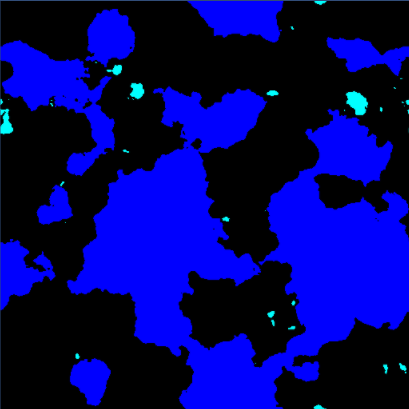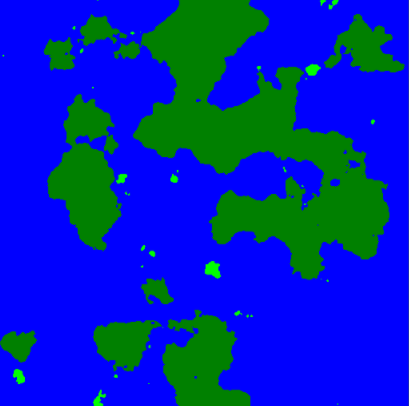The left side image, all land tiles are all black. The ocean tiles are blue, and the lake tiles are cyan.

The right side image, all water tiles are blue. Large land masses are dark green, and islands in light green.

As you can see, we now have a lot more information on our generated map, and it effectively answers all of the questions we set out to answer.

Source code for Part 2 can be found here on github.

Continue to Part 3 of this series.

# 15 Responses

## mcdoobiecommented on July 14, 2016 at 3:35 pmGreat tut, how did you generate the flood filled textures?

## mcdoobiecommented on July 21, 2016 at 3:06 pmYeah, I just cant see how you use the FloodFill functions in that script.

## admincommented on July 26, 2016 at 11:45 amThe FloodFill() function is called when loaded. That function does a complete floodfill on the entire map. While doing this, it creates two tile groups — one for water tiles and one for land based tiles.

These collections of tiles then represent the data we need to generate those flood filled textures. The TextureGenerator is used with this data to spit out the final images.

## GobbyWahpcommented on July 29, 2016 at 8:48 pmA little interested on where I can find this MathHelper. I checked out http://forum.unity3d.com/threads/released-math-helper.164801/ but unfortunately this has been removed from the Unity Store. Preferably I wouldn’t want to spend money to complete a tutorial but you did such a great job I just wanted to thank you and ask where I could find that package at.

## mcdoobiecommented on August 7, 2016 at 1:05 pmNow I understand,
Really good, thank you very much.

## Jonathancommented on February 8, 2018 at 1:32 pmCould you please explain me this part of the code?

float heightValue = (float)HeightMap.Get (nx, ny, nz);

Is that “Get” thing something of yours?

## admincommented on February 8, 2018 at 3:27 pmHeightMap.Get is part of the ImplicitFractal.cs class. This is from the AccidentalNoise library. On this line of code, we are sampling the data at coordinate (nx, ny, nz).

The code is part of the project, and can be found here:

https://github.com/jongallant/WorldGeneratorPart2/blob/master/Assets/Scripts/AccidentalNoise/Implicit/ImplicitFractal.cs

This is not my code, as explained in the article. It is part of the Accidental Noise library.

## Nolatcommented on June 27, 2018 at 8:58 amHi !

I don’t understand how you do to differentiate ocean and lake / land and islands

## pcfreak9000commented on February 2, 2019 at 7:26 pmHi. I am using this in a different context, so without the MapData object (I render the values directly to a texture). With this

float heightValue = (float)HeightMap.Get (nx, ny, nz, nw);

call I almost never get any values greater than 0 with the 4D cylinders. Is that normal?

## Charliecommented on February 5, 2019 at 6:18 pmJon –

Thank you for this tutorial – it is incredibly helpful. One quick question … you mention above:

It would be nice if we could determine a few things, such as:

Where are the Lakes?
Where are the Oceans?
Where are the Land Masses?
How big are each of these?

I understand how you identified the Water and Land TileGroup based on Collidable, but how did you isolate the Lakes and the Small Islands as shown in the last two textures?

I presume it must have something to do with Size, but I can’t quite figure out how you used FloodFill to achieve that.

## admincommented on February 22, 2019 at 4:45 pmThe floodfill creates groups of tiles. I simply used the Count of each group to determine it’s size. Then you can compare, or set an arbitrary size value as the cutoff to determine what is a lake or an ocean.
The tiletype determines if it is land or water.

## Sorethcommented on March 21, 2019 at 3:46 pmCan’t figure out why it don’t work but tried a lot and can’t have my map wrapping on Both Axis.

var c = noise(nx,ny,nz,nw);
var x1 = 0, x2 = 2;
var y1 = 0, y2 = 2;
var dx = x2 – x1;
var dy = y2 – y1;

// Sample noise at smaller intervals
var s = x / width;
var t = y / height;

// Calculate our 4D coordinates
nx = x1 + Math.cos (s*2*Math.PI) * dx/(2*Math.PI);
ny = y1 + Math.cos (t*2*Math.PI) * dy/(2*Math.PI);
nz = x1 + Math.sin (s*2*Math.PI) * dx/(2*Math.PI);
nw = y1 + Math.sin (t*2*Math.PI) * dy/(2*Math.PI);
var c = noise(nx,ny,nz,nw);

## Sorethcommented on March 22, 2019 at 8:31 amI figured out why it didn’t worked, the lib I use don’t care about the 4th argument …
Lost hours just because peoples create fake functions …
Nevermind, thanks for your tutorial